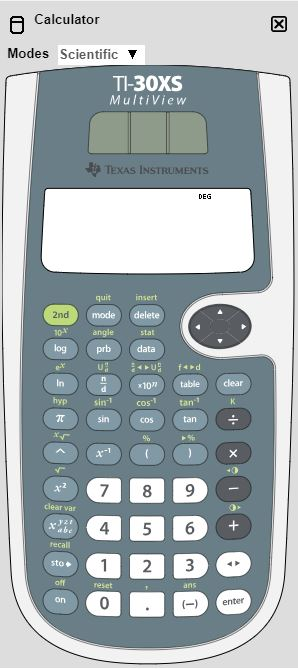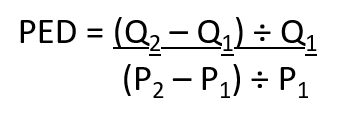# Prepare to Pass your ACCA exams: Week 2 – Get to know your calculator

In the exam room, you want to be able to rely on the answer your calculator has given you.

The problem is, if the information isn’t entered in the right way in your calculator, you’ll get an answer – but there is no way of telling whether it is the right one!

With complex computations appearing in exams such as Performance Management and Financial Management, it is really important to be comfortable and confident in using your calculator.

Therefore, in this week’s blog, I want to focus on my top three features available on a scientific calculator and show how you can use them to solve some of the tricky calculations in the exams.

In the computer-based exam you’ll have access to an online calculator and the ACCA uses the Texas TI-30XS MultiView Scientific Calculator:You will need to have a scientific calculator as you study the subject to be able to practise questions.

Remember you can take your own calculator into the exam, and it doesn’t have to be the ACCA’s make and model, but it must be non-programmable. You may already have a calculator from school, university or work that you prefer.  My old faithful calculator is the Casio fx-85GT PLUS but, of course, other calculators are available!

1. Switching between decimals and fractions

Try entering 36 ÷ 5 = into your calculator. What do you get? 7.2 or 365  ?

Your calculator will allow you to switch between giving the answer in the form of a decimal or a fraction depending on which your need.

Look out for a double arrow < > key (sometimes it appears as an S<>D key) which will allow you to toggle between the different formats.

1. Coping with “to the power of” questions

Consider a compound interest question in FM, where \$100 is invested at 10% and we need to find how much the investment is worth in 2 or 7 years’ time.

For the two-year question we can simply use the x2 button and enter:

100 x 1.10 x2 = and the answer is \$121

For any other time period, we need to use the “to the power of” function as indicated by the ^ sign (or sometimes it’s x with a small box). This simply gives you the opportunity to enter the relevant number of years.

We can therefore do our 7-year calculation as follows:

100 x 1.10 ^ 7 = and the answer is \$195

1. Managing bigger calculations

The Price Elasticity of Demand formula from PM is quite tricky:Let’s assume P1 is 400, P2 is 405, Q1 is 1,000 and Q2 is 950.

You could do the two separate calculations for the top and bottom parts of the formula i.e.:To save time we can make use of the fraction formula often shown as nd being the numerator divided by the denominator (or the Casio has it as one box divided by another).

Press it and you then have the ability to enter the top part and bottom part of the formula. Note to move between the two you’ll need to use the arrow up and down keys.

Let’s try doing that calculation again on our calculators:

nd and enter (950-1,000) ÷ 1,000 on the top

ARROW DOWN and enter (405 – 400) ÷ 400 on the bottom

RIGHT ARROW =